# Articles by PyShark

### Binomial Distribution and Binomial Test in Python

October 1, 2021 | PyShark

In this article we will explore binomial distribution and binomial test in Python. Table of contents Introduction What is a binomial distribution Create and plot... The post Binomial Distribution and Binomial Test in Python appeared first on PyShark.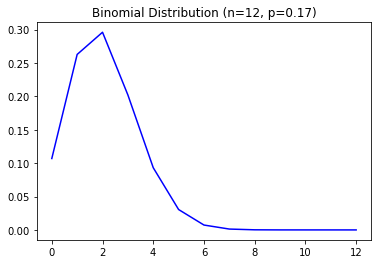### Test for Normality Using Python: Beginner’s Guide

September 5, 2021 | PyShark

In this tutorial we will explore how to test for normality using Python. Table of contents Introduction Sample data for normality testing Q-Q plot in... The post Test for Normality Using Python: Beginner’s Guide appeared first on PyShark.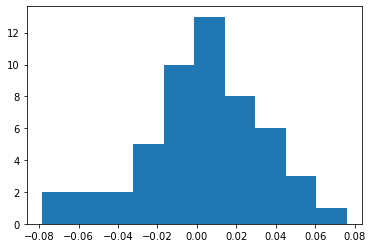### Kurtosis in Python

September 2, 2021 | PyShark

In this tutorial we will explore how to calculate kurtosis in Python. Table of contents Introduction What is kurtosis? How to calculate kurtosis? How to... The post Kurtosis in Python appeared first on PyShark.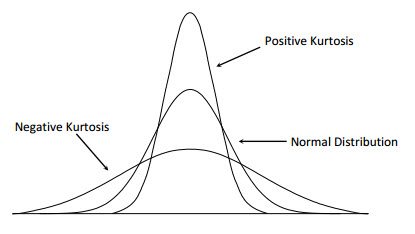### Skewness in Python

July 26, 2021 | PyShark

In this tutorial we will explore how to calculate skewness in Python. Table of contents Introduction What is skewness? How to calculate skewness? How to... The post Skewness in Python appeared first on PyShark.### Take Screenshot using Python

July 22, 2021 | PyShark

In this tutorial we will explore multiple ways of how to take a screenshot using Python. Table of contents Introduction Take screenshot with pyautogui Take... The post Take Screenshot using Python appeared first on PyShark.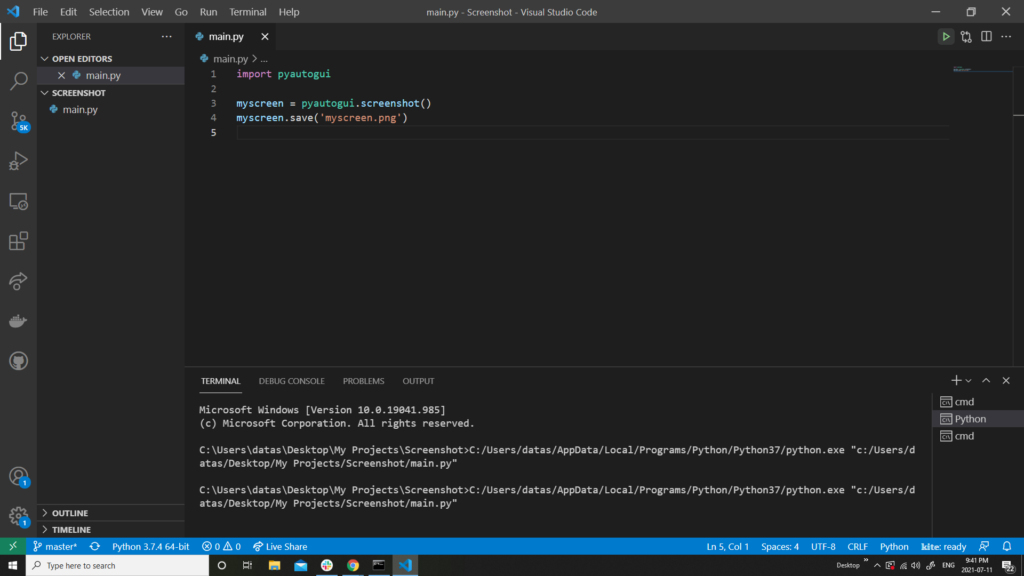### Inner (Nested) Classes in Python

July 6, 2021 | PyShark

In this tutorial we will explore what inner (nested) classes are and how to use them in Python. Table of contents What are inner (nested)... The post Inner (Nested) Classes in Python appeared first on PyShark. [...Read more...]

### Extract Table from PDF using Python

June 27, 2021 | PyShark

In this tutorial we will discuss how to extract table from PDF files using Python. Table of contents Introduction Sample PDF files Extract single table... The post Extract Table from PDF using Python appeared first on PyShark.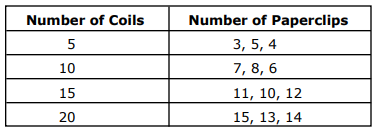### Davies-Bouldin Index for K-Means Clustering Evaluation in Python

June 2, 2021 | PyShark

In this tutorial we will explore the Davies-Bouldin index and its application to K-Means clustering evaluation in Python. Table of Contents Introduction Davies-Bouldin Index Step... The post Davies-Bouldin Index for K-Means Clustering Evaluation in Python appeared first on PyShark.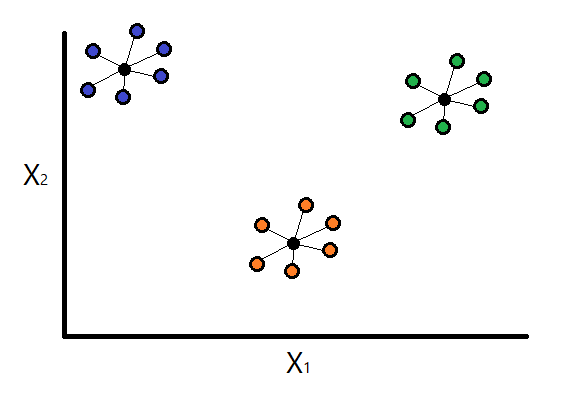### Convert Pandas DataFrame to NumPy Array in Python

May 2, 2021 | PyShark

In this article we will discuss how to convert Pandas DataFrame to NumPy array in Python. Table of Contents Introduction Create a sample Pandas DataFrame... The post Convert Pandas DataFrame to NumPy Array in Python appeared first on PyShark. [...Read more...]

### Calculate Factorial in Python

April 27, 2021 | PyShark

In this article we will discuss how to calculate a factorial in Python. Table of Contents Introduction Factorial formula Calculating factorial in Python Factorial functions... The post Calculate Factorial in Python appeared first on PyShark.